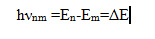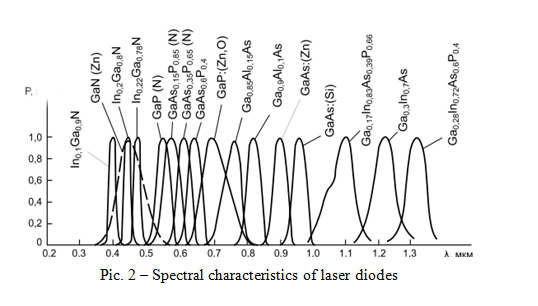Generic selectors
Exact matches only
Search in title
Search in content
Search in posts
Search in pages## One of the most important laser parameters is the generated radiation wavelength.

The laser application field depends on the laser wavelength. Lasers are used in various fields, such as: medicine, security and monitoring systems, manufacturing, etc. Why does a laser have this or that wavelength parameter? To understand that you need to refer to the physics of the radiation process.

Any type of radiation is characterized by the energy transportation from a radiating physical system to a receiving physical system. Every material body with a temperature above absolute zero Kelvin radiates or absorbs energy. Consequently, all the bodies, you deal with in every day life, continuously exchange energy. Therefore, radiation, being one of the qualitative varieties of energy, is a measure of the motion of matter – an objective reality that exists outside and independent of our consciousness. Radiation as a special form of matter has a dual nature – it possesses wave and corpuscular properties. Particles of matter under certain conditions can be converted into radiation, and radiation into particles of matter. The nature of radiation is associated with atomic and molecular processes in substances in all their aggregate states. The sources of radiation are atoms and molecules. The energy of these elementary emitters (harmonic oscillators) can only vary by jumps, divisible by a certain value of hν, constant for a given radiation frequency. M. Planck called this smallest dose of energy of the elementary emitter the quantum of energy and designated

E = hν,
where h is the Planck constant, h = 6.626 x 10^-34 J x s, ν is the frequency of the speed of light.
In turn, the radiation frequency is related to the wavelength as
ν = c / λ,

where c = 2.998 * 10^8 m / s – the speed of light.
(where h – the Planck constant, h=6,626 x 10^−34 J x s)

In accordance with the laws of quantum mechanics, the electron energy bound in an atom, as well as the atom energy, cannot take arbitrary amount. It has a certain discrete series of values E0, E1, E2, …, En, called energy levels. The level with the lowest energy of the atom E0 is called the ground level, the remaining levels E1, E2, …, En, corresponding to the higher energy of the atom, are excited. Under certain conditions, an electron can make an abrupt transition from one energy level to another. With similar quantum transitions between the energy levels m and n, the atom emits or absorbs electromagnetic waves in certain portions – energy quanta (photons hνnm). The frequencies of electromagnetic waves in quantum transitions are determined by N. Bohr’s second postulate:This equation connects the wave and quantum properties of radiation. When the photon hνnm is absorbed, the energy of the atom increases by this amount, and the quantum transition of the atom from the lower energy level Em to the upper En occurs, and when the photon hνnm is emitted, the quantum transition of the atom to the lower level Em occurs at a frequency νnm.

If we refer the above to a semiconductor laser, then the considered energy is the energy difference in the semiconductor between the conduction bands and the valence band. A laser diode, as well as any diode-semiconductor, which is characterized by the presence of a valence band, a conduction band, and a forbidden band. These zones characterize the distribution of electrons on the orbitals of the atom. At absolute zero, there are no electrons in the conduction band. Increasing of the temperature increases the energy of the system, this contributes to the thermal generation of charge carriers: electrons that have received energy equal to or greater than the width of the forbidden band are transferred to the conduction band and become free. The formation of a free electron entails the formation of an electron-hole pair – a vacant place is formed at the site of the released electron. A pictorial diagram is shown in Figure 1.Pic. 1 – Generation (bottom up) and recombination (top down) of electrons

Electrons and holes formed as a result of generation, perform thermal motion in a semiconductor crystal for a certain period of time, called the lifetime. The transition of a free electron back to the lower level is called recombination. This transition is of particular interest, because in this case the electron loses energy and this transition can be radiative with the energy of the emitted photon E = hν, or nonradiative with the conversion of energy into heat. The plurality of the radiative transitions at a given period of time is laser radiation.
Thus, the frequency of light or the wavelength of the laser radiation is determined by the width of the forbidden band in a semiconductor crystal.

In the above equation, there are two constants (h, c) and two proportional quantities (E, λ). That is, the wavelength can be changed by changing the energy of the forbidden band. Is it possible to make the structure of the conductor change the width of the forbidden zone? It is possible. You need to introduce a certain number of foreign atoms. This will lead to a significant change in its electrical properties: impurities contribute to the narrowing of the band gap due to the appearance of additional energy levels. Thus, decrease in the energy of the band gap promotes the growth of the wavelength. Figure 2 shows the spectral characteristics of laser diodes made of semiconductor materials of Ga compounds.5.00/5 Article rating 11 reviewsLoading...
Posted in Misc, World developments

1. (please, enter a valid email)1. (please, enter a valid email)